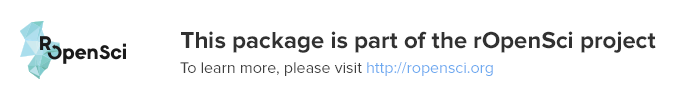# README.md In plotly: Create Interactive Web Graphics via 'plotly.js'An R package for creating interactive web graphics via the open source JavaScript graphing library plotly.js.

## Installation

Install from CRAN:

``````install.packages("plotly")
``````

Or install the latest development version (on GitHub) via devtools:

``````devtools::install_github("ropensci/plotly")
``````

## Getting started

### Web-based ggplot2 graphics

If you use ggplot2, `ggplotly()` converts your static plots to an interactive web-based version!

``````library(plotly)
g <- ggplot(faithful, aes(x = eruptions, y = waiting)) +
stat_density_2d(aes(fill = ..level..), geom = "polygon") +
xlim(1, 6) + ylim(40, 100)
ggplotly(g)
``````By default, `ggplotly()` tries to replicate the static ggplot2 version exactly (before any interaction occurs), but sometimes you need greater control over the interactive behavior. The `ggplotly()` function itself has some convenient “high-level” arguments, such as `dynamicTicks`, which tells plotly.js to dynamically recompute axes, when appropriate. The `style()` function also comes in handy for modifying the underlying trace attributes (e.g. hoveron) used to generate the plot:

``````gg <- ggplotly(g, dynamicTicks = "y")
style(gg, hoveron = "points", hoverinfo = "x+y+text", hoverlabel = list(bgcolor = "white"))
``````Moreover, since `ggplotly()` returns a plotly object, you can apply essentially any function from the R package on that object. Some useful ones include `layout()` (for customizing the layout), `add_traces()` (and its higher-level `add_*()` siblings, for example `add_polygons()`, for adding new traces/data), `subplot()` (for combining multiple plotly objects), and `plotly_json()` (for inspecting the underlying JSON sent to plotly.js).

The `ggplotly()` function will also respect some “unofficial” ggplot2 aesthetics, namely `text` (for customizing the tooltip), `frame` (for creating animations), and `ids` (for ensuring sensible smooth transitions).

### Using plotly without ggplot2

The `plot_ly()` function provides a more direct interface to plotly.js so you can leverage more specialized chart types (e.g., parallel coordinates or maps) or even some visualization that the ggplot2 API won’t ever support (e.g., surface, mesh, trisurf, etc).

``````plot_ly(z = ~volcano, type = "surface")
``````To learn more about special features that the plotly R package provides (e.g., client-side linking, shiny integration, editing and generating static images, custom events in JavaScript, and more), see https://plotly-r.com. You may already be familiar with existing plotly documentation (e.g., https://plot.ly/r/), which is essentially a language-agnostic how-to guide for learning plotly.js, whereas https://plotly-r.com is meant to be more wholistic tutorial written by and for the R user. The package itself ships with a number of demos (list them by running `demo(package = "plotly")`) and shiny/rmarkdown examples (list them by running `plotly_example("shiny")` or `plotly_example("rmd")`). Carson also keeps numerous slide decks with useful examples and concepts.

## Contributing

Please read through our contributing guidelines. Included are directions for opening issues, asking questions, contributing changes to plotly, and our code of conduct.## Try the plotly package in your browser

Any scripts or data that you put into this service are public.

plotly documentation built on Nov. 8, 2019, 1:07 a.m.